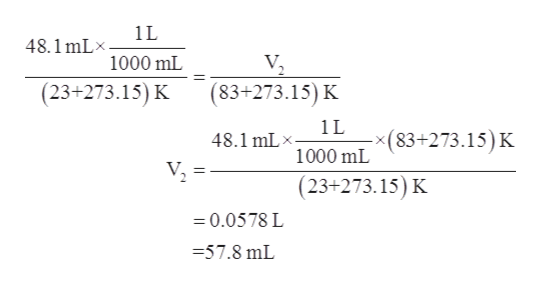# A 48.1 mL sample of gas in a cylinder is warmed from 23 ∘C to 83 ∘C.What is its volume at the final temperature? (Assume constant pressure.)

Question
49 views

A 48.1 mL sample of gas in a cylinder is warmed from 23 ∘C to 83 ∘C.

What is its volume at the final temperature? (Assume constant pressure.)

check_circle

Step 1

Given information:

Temperature of the gas at initial stage (T1) = 23 °C

Volume at initial stage (V1) = 48.1 mL

Temperature at final stage (T2) = 83 °C

Step 2

Charles Law states that at constant pressure , the volume of a sample is directly proportional to the temperature.

The expression for Charles Law is represented as follows:

Step 3

Plugging the values of T1, V1, and T2 in Charles La...help_outlineImage Transcriptionclose1L 48.1 mLx V 1000 mL (23+273.15) K (83+273.15) K 1L (83+273.15) K 48.1 mL 1000 mL (23+273.15) K =0.0578 L -57.8 mL fullscreen

### Want to see the full answer?

See Solution

#### Want to see this answer and more?

Solutions are written by subject experts who are available 24/7. Questions are typically answered within 1 hour.*

See Solution
*Response times may vary by subject and question.
Tagged in

### Gas laws